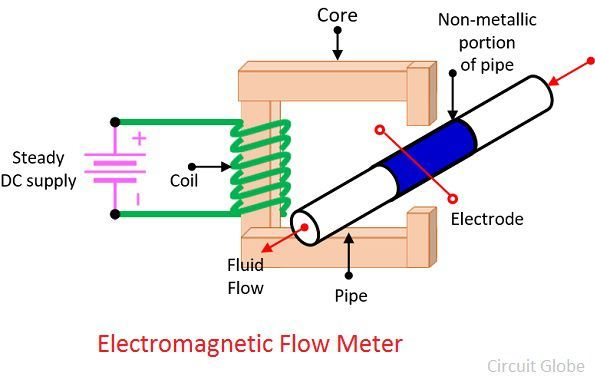# Electromagnetic Flow Meter

Definition: The electromagnetic flow meter is a device used for measuring the flow of the liquid when it passes through the pipeline. Or we can say that the electromagnetic flowmeter use for measuring the flow rate of the electrically conducting fluid. The electrically conductive liquid means the liquid allows the current to pass through it.

The electromagnetic flowmeters work on the principle that the obstruction is created in the path of the liquid and the pressure of the liquid induces the voltage across the coil.

## Working Principle of Electromagnetic Flow Meter

The electromagnetic flowmeter works on the principle of Faraday’s Law of electromagnetic induction. This law states that when the conductive liquid passes through the magnetic field, the voltage induces across the conductor. The magnitude of the voltage is directly proportional to the velocity, length of the conductor and the strength of the magnetic field.The magnetic field is generated by the coil which is mounted on the external metallic body of the pipe. The liquid acts as a conductor and when passes through the magnetic field induce the voltage across the coil. The magnitude of the voltage depends on the velocity of the liquid.

## Construction of Electromagnetic Flow Meter

The electromagnetic flow meter consists the electrically insulated pipe made of fibre. Electrodes placed opposite to each other, magnetic coil placed on the pipe for generating the magnetic field etc. The insulated pipe carries the liquid whose flow needs to be measured.The electromagnet is placed around the insulated pipe. This electromagnet induces the magnetic field around the pipe. The arrangement is similar to the conductor moving in the magnetic field. The voltage is induced across the coil because of the flow of the liquid. The induces voltage is expressed as,Where, v – velocity of conductor (flow); m/s
l – length of conductor = diameter of pipe ;m
B = flux X density ; wb/m2

If the magnetic field around the pipe remains constant than the generating voltage is proportional to the velocity of the fluid.

### Advantages of Electromagnetic Flow Meter

1. The output voltage of the electromagnetic flow meter is proportional to the flow rate of the liquid.
2. The output is uninfluenced by the varying characteristic of liquid like viscosity, pressure, temperature etc.
3. The electromagnetic flow meter can measure the flow of slurries, greasy, and can handle the corrosive fluid liquids.
4. It is used as a bidirectional meter.
5. The extremely low flow rate can also be measured by the electromagnetic flow meter.

### Disadvantages of Electromagnetic Flow Meter

1. The electromagnetic flow meter has low accuracy.
2. It is heavy and extremely large in size.

The electromagnetic flow meter is also known as the magmeter.